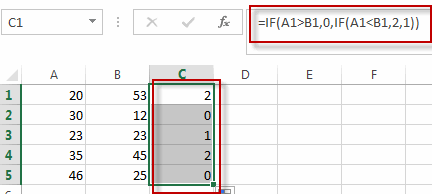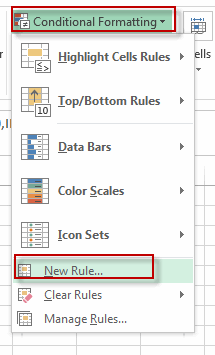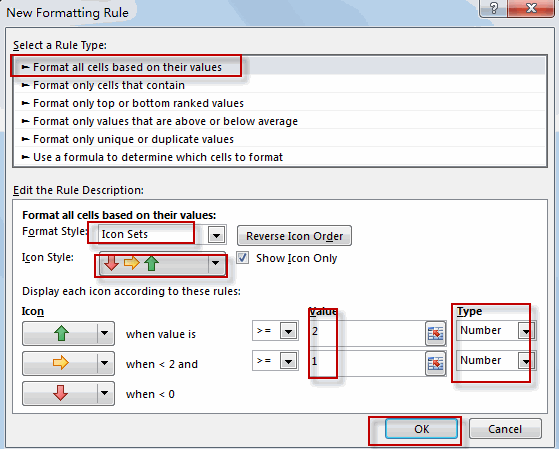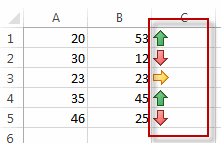# Comparing Columns Using Conditional Formatting Icon Sets

This post will guide you how to compare the adjacent cells in the different columns using Conditional Formatting Icon Sets in Excel. How do I compare cells in the adjacent rows using Conditional Formatting Icon Sets in Excel. How to compare Columns or rows using Conditional Formatting Icon Sets to show increase or decrease status in your current worksheet. How to use the conditional formatting feature to show trend arrows when comparing the adjacent columns or rows.

Assuming that you have a list of data A1:B5, and you want to compare cells in Column A with Column B, if the value in Cells in Column B is greater than the value of cells in Column A, then showing an up arrow Icon in the Column C; If the value of cells in Column B is equal to the value in Column A, then showing an right arrow icon in column C; If the value of cells in Column B is less than the value in Column A, then showing an down arrow icon in Column C. How to achieve it.

## Comparing Columns Using Conditional Formatting Icon Sets

To compare the adjacent columns using conditional formatting icon sets in excel, you need to do the following steps:

#1 type this formula into the formula box of the first cell in column C, and drag the AutoFill Handler over other cells to apply this formula.

=IF(A1>B1,0,IF(A1<B1,2,1))This formula will compare the adjacent cells in Column A and B.

#2 go to HOME tab, Click Conditional Formatting command under Styles group. and select New Rule from the popup menu list. The New Formatting Rule dialog box will appear.#3 click Format all cells based on their values option from the Select a Rule Type list box. and select Icon Sets value from the drop-down list box of Format Style. Select 3 Arrows icon from the drop-down list of Icon Style. Check Show Icon Only option, and then type the value 2 when the Icon is up arrow icon. and type the value 1 when icon is right icon. Click Ok button.#4 Let’s see the result.### Related Functions

• Excel IF function
The Excel IF function perform a logical test to return one value if the condition is TRUE and return another value if the condition is FALSE. The IF function is a build-in function in Microsoft Excel and it is categorized as a Logical Function.The syntax of the IF function is as below:= IF (condition, [true_value], [false_value])….

Related Posts
If Cell is This Value or That Value

IF function is frequently used in Excel worksheet to return you expect “true value” or “false value” based on the result of logical test. If you want to see if a cell is A or B, and if one of ...

If Value is Greater Than A Certain Value

IF function is frequently used in Excel worksheet to return you expect “true value” or “false value” based on the logical test result. If you want to see if a value in one cell is greater than a specific value, ...

If Cell is Not Blank

IF function is frequently used in Excel worksheet to return you expect “true value” or “false value” based on the result of created logical test. If you want to see if a cell is blank or not, and leave some ...

If Cell is Blank

IF function is frequently used in Excel worksheet to return you expect “true value” or “false value” based on the result of created logical test. If you want to see if a cell is blank or not, and leave some ...

If Cell Equals Certain Text String

IF function is frequently used in Excel worksheet to return you expect “true value” or “false value” based on the result of created logical test. If you want to see if cell equals a certain text string like “Win”, you ...

If Cell Contains Either Text1 or Text2

IF function is frequently used in Excel worksheet to return “true value” or “false value” based on the logical test result. If you want to see if cell contains certain substring1 like “abc” or substring2 like “def”, and returns true ...

If Cell Contains Certain Text OR Equals Certain Text

IF cell equals certain text IF function is frequently used in Excel worksheet to return “true value” or “false value” based on the logical test result. If you want to test values to see if they equal certain text like ...

Fix #N/A Error For VLOOKUP From Another Sheet

This post will show you how to fix the #N/A error why it occurs when you extract values from another sheet using VLOOKUP function in Excel 2016,2013,2010 or other Excel versions. How can you correct a #N/A error in VLOOKUP ...

How to Average Only Positive or Negative Numbers of a Range

Suppose both positive numbers and negative numbers exist in a table. If we want to know the average of only positive numbers in this table, we can create a formula to get average of all positive numbers with all negative ...

VLOOKUP with Two Lookup Tables

VLOOKUP is one of the key functions among all lookup & reference functions in Excel. Today we will show you the application of VLOOKUP function when there are two lookup tables. EXAMPLE Table1 and table2 record the rates of Y2020 ...

Sidebar